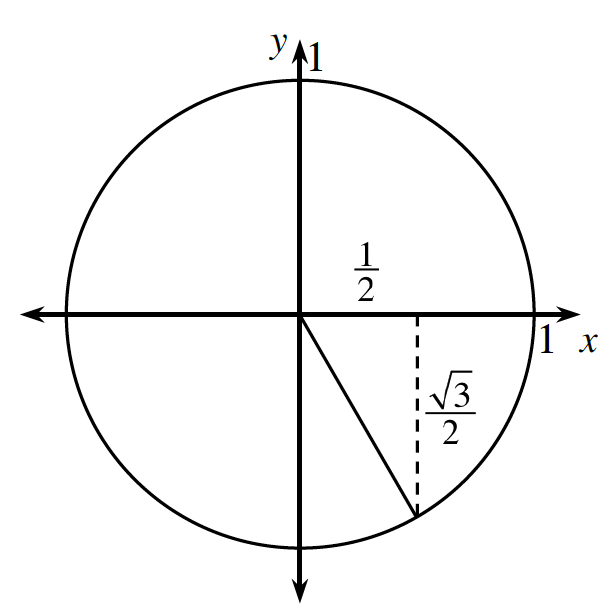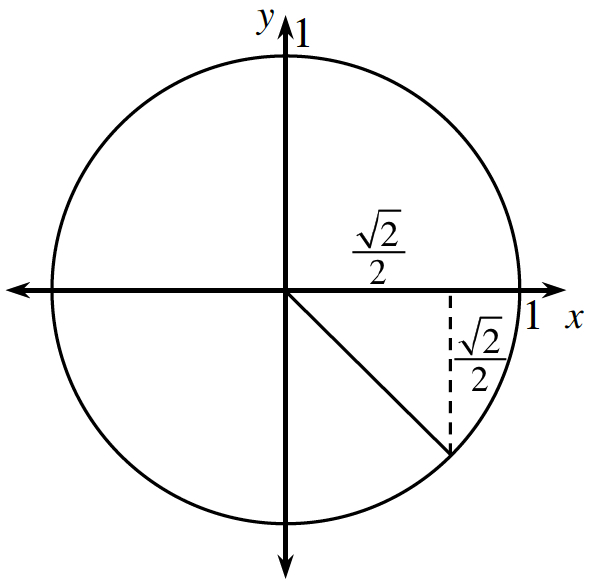### Home > PC3 > Chapter 5 > Lesson 5.1.1 > Problem5-9

5-9.

Evaluate each of the following trigonometric expressions without a calculator.1. $\sin\left(\frac{5\pi}{3}\right)$1. $\cos\left(-\frac{\pi}{4}\right)$1. $\tan\left(\frac{\pi}{2}\right)$

What is the slope of the line associated with an angle of $\frac{\pi}{2}$?